## Convolutional neural network processing sequence for Python deep learning

CDFMLR 2021-08-09 15:10:09
convolutional neural network processing sequence

This is my participation 8 The fourth of the yuegengwen challenge 9 God , Check out the activity details ：8 Yuegengwen challenge

# Deep Learning with Python

This article is for me to learn 《Deep Learning with Python》( The second edition ,François Chollet Writing ) One of a series of notes written when . The content of the article is from Jupyter notebooks Turn into Markdown Of , You can go to GitHub or Gitee Find the original `.ipynb` The notebook .

You can go to This website reads the original version of the book online ( english ). The author of this book also gives the supporting Jupyter notebooks.

This paper is about The first 6 Chapter Deep learning is used for text and sequences (Chapter 6. Deep learning for text and sequences) The notes .

## 6.4 Sequence processing with convnets

Using convolutional neural network to process sequence

Convolutional neural network can make effective use of data , Extracting local features , Will represent modularity . Because of this special effect ,CNN Not only good at dealing with computer time problems , It can also deal with sequence problems efficiently , On some sequence problems ,CNN The effect of 、 The efficiency can even exceed RNN.

It is different from two-dimensional image processing Conv2D, Time series are one-dimensional , So we need to use one-dimensional convolutional neural network to deal with .

### One dimensional convolution of sequence data 、 Pooling

Similar to two-dimensional convolution , One dimensional convolution extracts local fragments from sequences （ Subsequence ）, Then do the same transformation for each fragment . A one-dimensional convolution window is a one-dimensional window on the time axis . The nature of the operation can guarantee , Patterns learned in one location can be recognized later in other locations （ It has time translation invariance ）.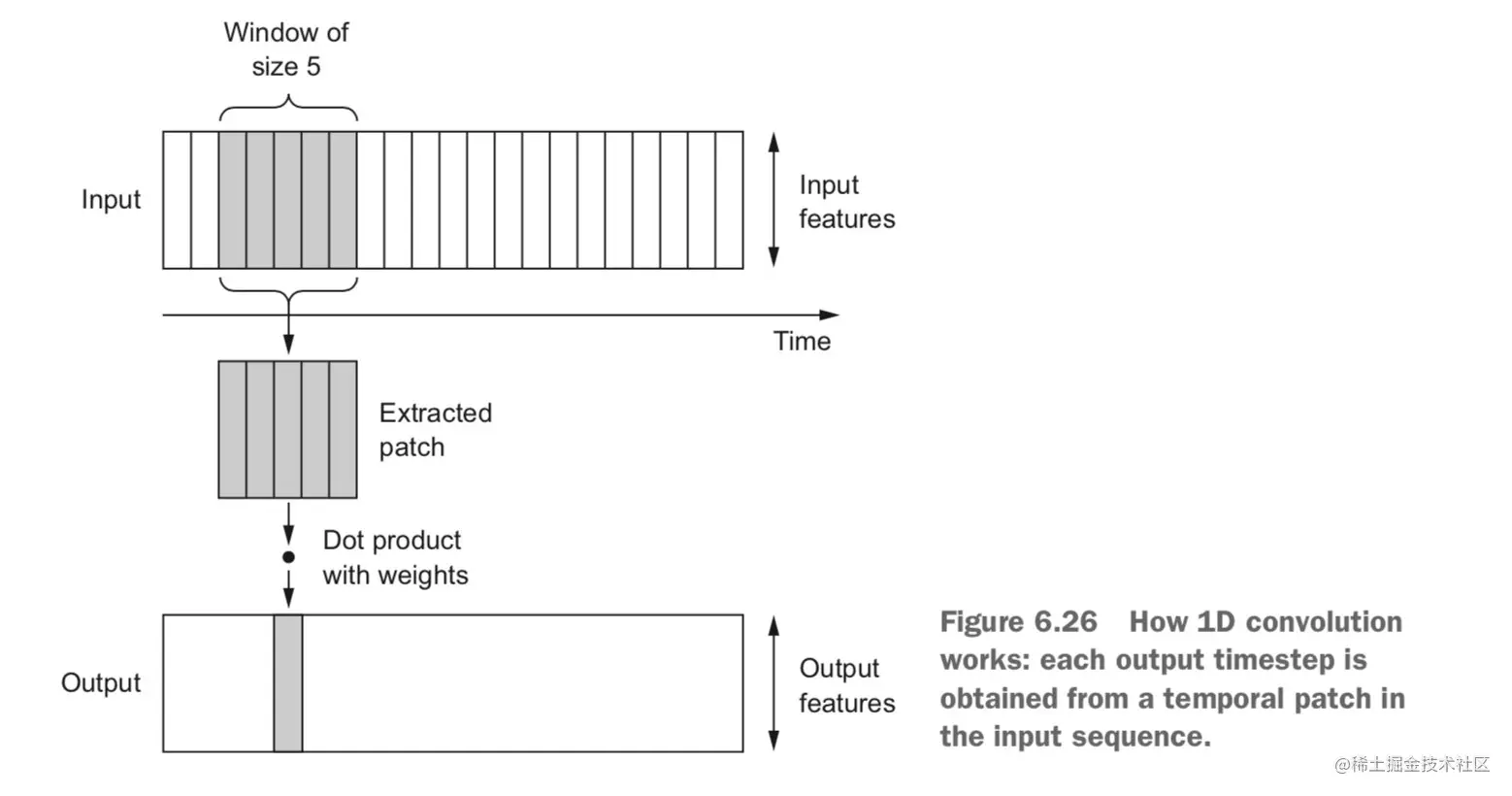One dimensional pooling operation , It is also similar to two-dimensional pooling operation ： Extract a one-dimensional fragment from the input , Output the maximum value ( Maximum pooling ) Or average ( The average pooling ). This operation is also used to reduce the length of data ( Do sub sampling ).

### Realize one-dimensional convolutional neural network

stay Keras in , One dimensional convolutional neural network Conv1D Layer to represent . Usage and Conv2D Is very similar , It receives a shape of `(samples, time, features)` The input of , Return is still in this shape . Be careful , Its window is in time Upper , The second axis of the input .Conv2D Our windows are usually 3x3、5x5 In this way , Corresponding Conv1D in , We usually take 7 or 9 The window size of .

Often , We will all Conv1D Layer and the MaxPooling1D Layers stacked together , At the end of all convolution pooling stacks , Then a global pooling operation or flattening operation .

Or to IMDB For example ：

``````from tensorflow.keras.datasets import imdb
from tensorflow.keras.preprocessing import sequence
max_features = 10000
max_len = 500
(x_train, y_train), (x_test, y_test) = imdb.load_data(num_words=max_features)
print(len(x_train), 'train sequences')
print(len(x_test), 'test sequences')
print('x_train shape:', x_train.shape)
print('x_test shape:', x_test.shape)
Copy code ``````

obtain ：

25000 train sequences

25000 test sequences

x_train shape: (25000, 500)

x_test shape: (25000, 500)

``````# stay IMDB A simple one-dimensional convolutional neural network is trained and evaluated
from tensorflow.keras.models import Sequential
from tensorflow.keras import layers
from tensorflow.keras.optimizers import RMSprop
model = Sequential()
model.summary()
model.compile(optimizer=RMSprop(lr=1e-4),
loss='binary_crossentropy',
metrics=['acc'])
history = model.fit(x_train, y_train,
epochs=10,
batch_size=128,
validation_split=0.2)
plot_acc_and_loss(history)
Copy code ``````

Get the model structure ：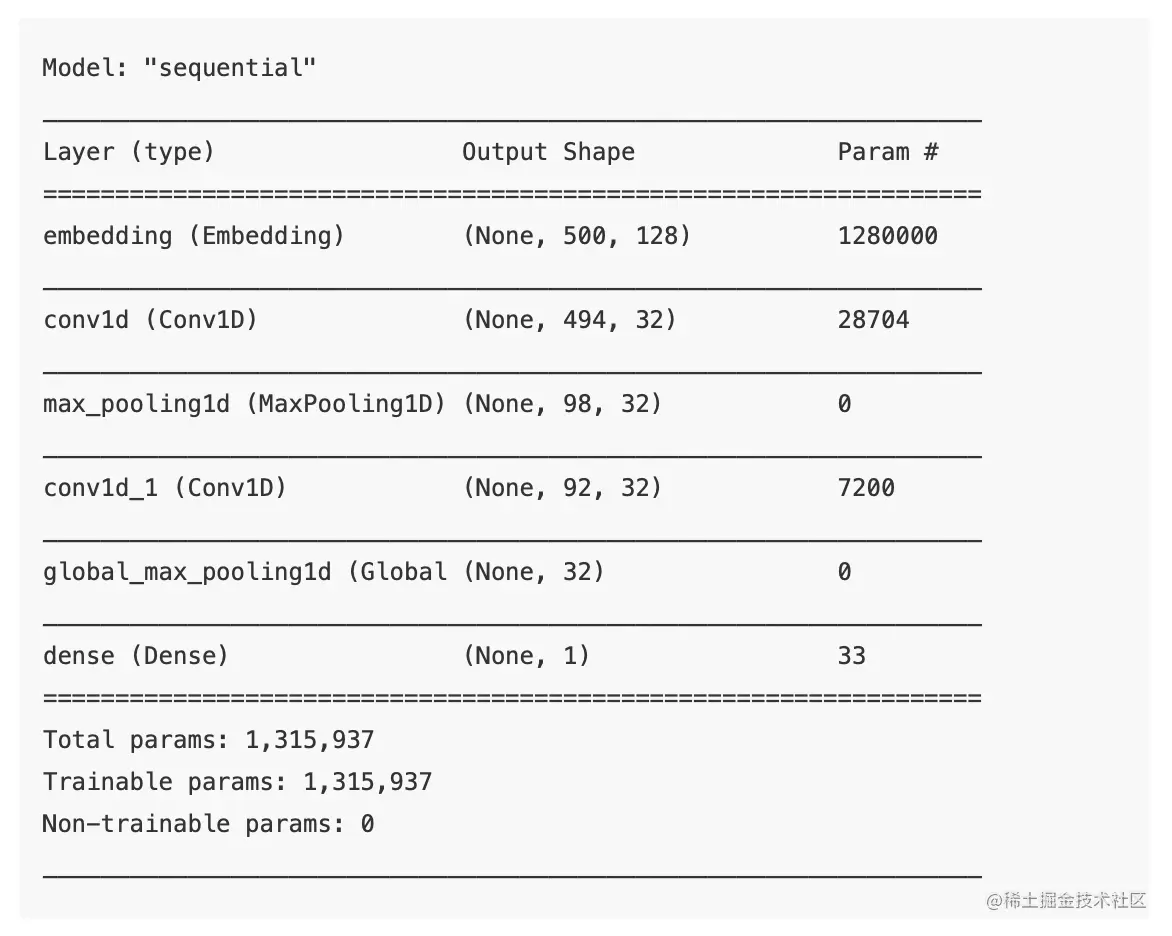Training process curve ：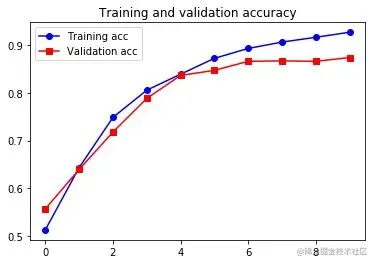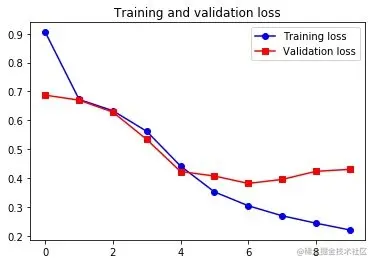Although the result is slightly better than RNN Bad , But it's pretty good , And training is better than LSTM fast .

### combination CNN and RNN Handle long sequences

One dimensional convolutional neural network divides the sequence into segments to learn , Insensitive to chronological order . So for those problems where the order of the sequence has a significant impact ,CNN Not as good as RNN. for example , Jena dataset ( Temperature forecast ) problem ：

First , Prepare the data ：

``````import os
import numpy as np
data_dir = "/CDFMLR/Files/dataset/jena_climate"
fname = os.path.join(data_dir, 'jena_climate_2009_2016.csv')
f = open(fname)
f.close()
lines = data.split('\n')
lines = lines[1:]
float_data = np.zeros((len(lines), len(header) - 1))
for i, line in enumerate(lines):
values = [float(x) for x in line.split(',')[1:]]
float_data[i, :] = values
mean = float_data[:200000].mean(axis=0)
float_data -= mean
std = float_data[:200000].std(axis=0)
float_data /= std
def generator(data, lookback, delay, min_index, max_index, shuffle=False, batch_size=128, step=6):
if max_index is None:
max_index = len(data) - delay - 1
i = min_index + lookback
while 1:
if shuffle:
rows = np.random.randint(
min_index + lookback, max_index, size=batch_size)
else:
if i + batch_size >= max_index:
i = min_index + lookback
rows = np.arange(i, min(i + batch_size, max_index))
i += len(rows)
samples = np.zeros((len(rows),
lookback // step,
data.shape[-1]))
targets = np.zeros((len(rows),))
for j, row in enumerate(rows):
indices = range(rows[j] - lookback, rows[j], step)
samples[j] = data[indices]
targets[j] = data[rows[j] + delay]
yield samples, targets
lookback = 1440
step = 6
delay = 144
batch_size = 128
train_gen = generator(float_data,
lookback=lookback,
delay=delay,
min_index=0,
max_index=200000,
shuffle=True,
step=step,
batch_size=batch_size)
val_gen = generator(float_data,
lookback=lookback,
delay=delay,
min_index=200001,
max_index=300000,
step=step,
batch_size=batch_size)
test_gen = generator(float_data,
lookback=lookback,
delay=delay,
min_index=300001,
max_index=None,
step=step,
batch_size=batch_size)
val_steps = (300000 - 200001 - lookback) // batch_size
test_steps = (len(float_data) - 300001 - lookback) // batch_size
Copy code ``````

A simple one-dimensional convolutional neural network is trained and evaluated on Jena data set ：

``````from tensorflow.keras.models import Sequential
from tensorflow.keras import layers
from tensorflow.keras.optimizers import RMSprop
model = Sequential()
input_shape=(None, float_data.shape[-1])))
model.compile(optimizer=RMSprop(), loss='mae')
history = model.fit_generator(train_gen,
steps_per_epoch=500,
epochs=20,
validation_data=val_gen,
validation_steps=val_steps)
plot_acc_and_loss(history)
Copy code ``````

Training process curve ：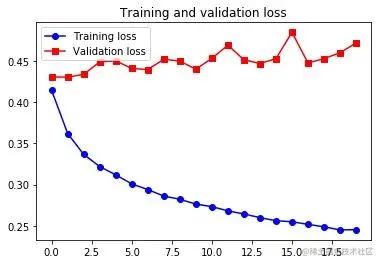This is not as good as the common sense method we use , It can be seen that sequence information is still very key to this problem . In order to learn sequential information , At the same time, the speed and weight of convolutional neural network are maintained , We can use it together CNN and RNN.

We can do it in RNN Use... In the front Conv1D. For very long sequences ( For example, it contains thousands of time steps ), Direct use RNN Processing is too slow 、 Can't even handle . stay RNN Add some... To the front Conv1D You can convert an overly long input sequence ( Down sampling ) A shorter sequence of high-level features , And then use RNN To deal with order sensitive information that can be learned .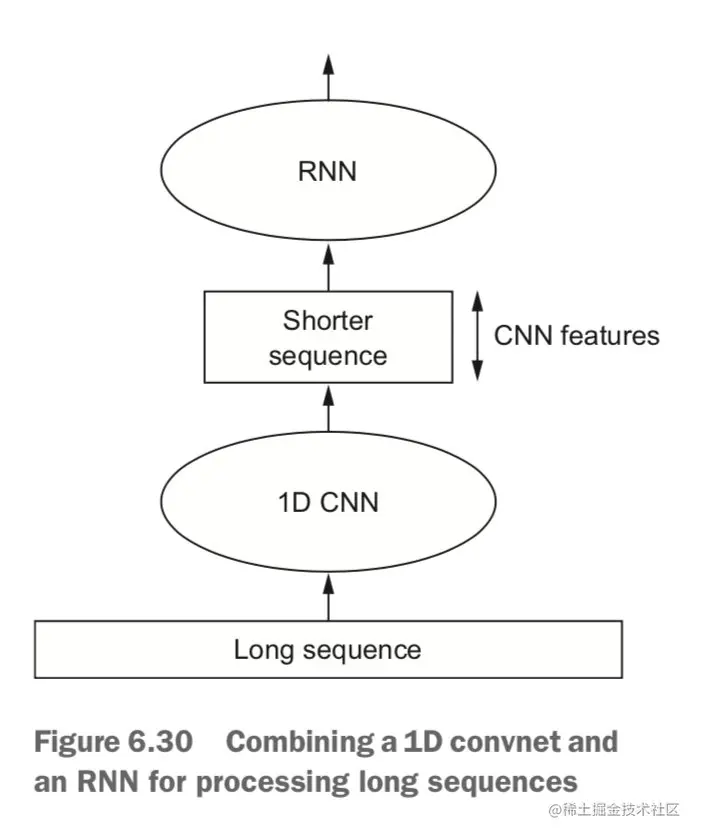Let's use this method to predict the temperature again , Because this method can learn longer sequences , We can let the network view earlier data ( Increase the size of the data generator lookback Parameters ), You can also let the network view time series with higher resolution （ Reduce the of the generator step Parameters ）：

``````step = 3 # 30 Minute by minute , It's half shorter than before
lookback = 720
delay = 144
train_gen = generator(float_data,
lookback=lookback,
delay=delay,
min_index=0,
max_index=200000,
shuffle=True,
step=step)
val_gen = generator(float_data,
lookback=lookback,
delay=delay,
min_index=200001,
max_index=300000,
step=step)
test_gen = generator(float_data,
lookback=lookback,
delay=delay,
min_index=300001,
max_index=None,
step=step)
val_steps = (300000 - 200001 - lookback) // 128
test_steps = (len(float_data) - 300001 - lookback) // 128
Copy code ``````

To build the network , use Conv1D + GRU：

``````model = Sequential()
input_shape=(None, float_data.shape[-1])))
model.summary()
model.compile(optimizer=RMSprop(), loss='mae')
history = model.fit_generator(train_gen,
steps_per_epoch=500,
epochs=20,
validation_data=val_gen,
validation_steps=val_steps)
plot_acc_and_loss(history)
Copy code ``````

Model structure ：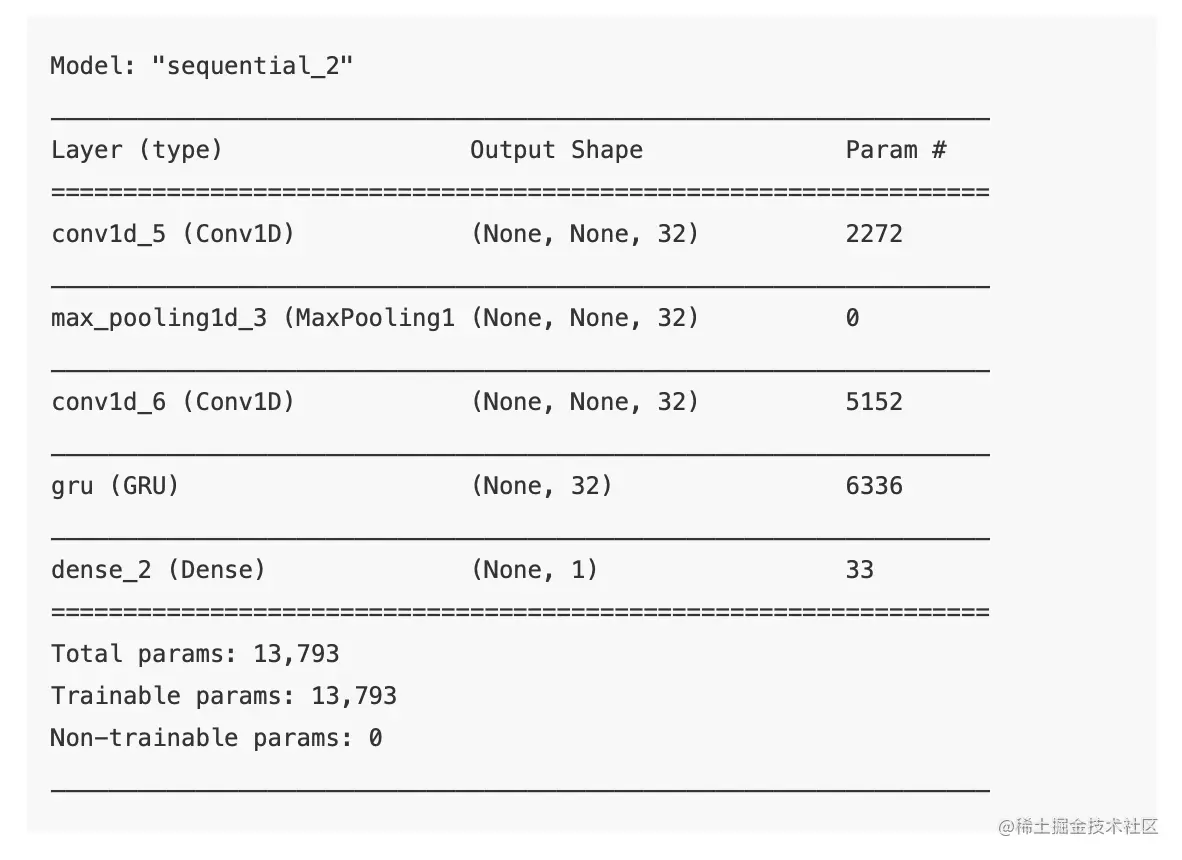Training process curve ：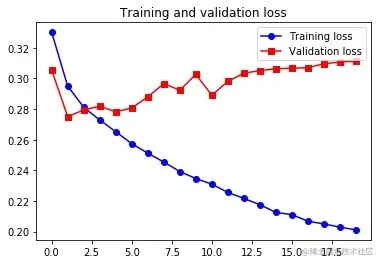In terms of verification loss , This architecture is less effective than just regularization GRU, But much faster . It looks at twice the amount of data , In this case, it may not be very useful , But for other datasets, it can be very important .

By("CDFMLR", "2020-08-14")

https://pythonmana.com/2021/08/20210809150912491k.html